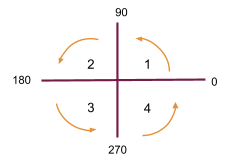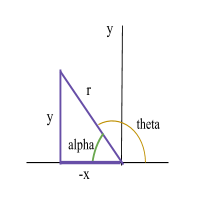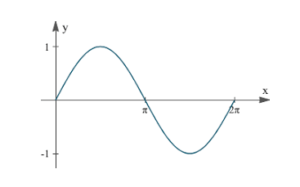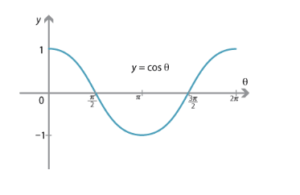# Sin, Cos and Tan

Contents

### Summary

•$sin\theta \quad =\quad \frac { opposite }{ hypotenuse }$
•$cos\theta \quad =\quad \frac { adjacent }{ hypotenuse }$
•$tan\theta \quad =\quad \frac { opposite }{ adjacent }$$sin\theta \quad =\quad \frac { opposite }{ hypotenuse }$$cos\theta \quad =\quad \frac { adjacent }{ hypotenuse }$$tan\theta \quad =\quad \frac { opposite }{ adjacent }$

We now consider angles in cartesian plane. We divide the plane into four quadrants in the anticlockwise sense as shown in the diagram. An angle formed, when the line OA rotates anticlockwise is taken to be positive, while clockwise rotation gives negative angles.The angle$\alpha$  is always acute regardless of the value of$\theta$  and is called the ”associated acute angle”.

In the first quadrant,$\theta$ is acute and all values of x, y and r are positive, therefore all the trigonometric ratios are positive.$cos\theta \quad =\quad \frac { x }{ r } \quad >\quad 0,$$cos\theta \quad =\quad \frac { x }{ r } \quad >\quad 0,$$tan\theta \quad =\quad \frac { y }{ x } \quad >\quad 0$

Acute angle$\alpha \quad =\quad 180\quad -\quad \theta$Hence:$sin\alpha \quad =\quad \frac { +y }{ r } \quad >\quad 0,\quad (+)$$cos\alpha \quad =\quad \frac { -x }{ r } \quad <\quad 0,\quad (-)$$tan\alpha \quad =\quad \frac { y }{ -x } \quad <\quad 0,\quad (-)$

In this quadrant angle$\alpha$  and$\theta$  are related by$\theta \quad =\quad 180\quad +\quad \alpha$Hence:$sin\alpha \quad =\quad \frac { -y }{ r } \quad <\quad 0,\quad (-)$$cos\alpha \quad =\quad \frac { -x }{ r } \quad <\quad 0,\quad (-)$$tan\alpha \quad =\quad \frac { -y }{ -x } \quad >\quad 0,\quad (+)$

In this quadrant angle$\alpha$  and$\theta$  are related by$\theta \quad =\quad 360\quad -\quad \alpha$Hence:$sin\alpha \quad =\quad \frac { -y }{ r } \quad <\quad 0,\quad (-)$$cos\alpha \quad =\quad \frac { x }{ r } \quad >\quad 0,\quad (+)$$tan\alpha \quad =\quad \frac { -y }{ x } \quad <\quad 0,\quad (-)$

The signs of the trigonometric ratios in the four quadrants can be summarised in the following “ACTS” diagram.### Graphs of sin, cos and tan

• sin$\theta$• cos$\theta$• tan$\theta$